# Exponentials & Logarithms: College Algebra Lesson Plans Chapter Exam

Exam Instructions:

Choose your answers to the questions and click 'Next' to see the next set of questions. You can skip questions if you would like and come back to them later with the yellow "Go To First Skipped Question" button. When you have completed the practice exam, a green submit button will appear. Click it to see your results. Good luck!

### Page 1

#### Question 1 1. Evaluate the logarithm below: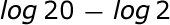#### Question 2 2. Evaluate the logarithm below: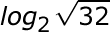#### Question 3 3. Evaluate the following.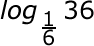#### Question 4 4. Solve the exponential equation below for x: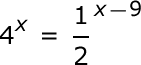### Page 2

#### Question 7 7. Expand the following logarithm: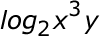#### Question 9 9. Which logarithmic property is shown below?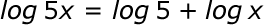#### Question 10 10. Solve, to 2 decimal places, the exponential equation below for x: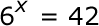### Page 3

#### Question 12 12. Solve the exponential equation below for x: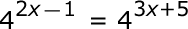#### Question 15 15. Evaluate the logarithm below to 2 decimal places.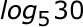### Page 4

#### Question 17 17. Evaluate the following.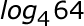#### Question 18 18. Solve the following equation for x.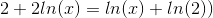#### Question 19 19. Solve the exponential equation below for x: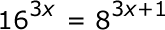#### Question 20 20. Condense the logarithm below: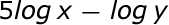### Page 5

#### Question 23 23. Evaluate the following.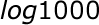### Page 6

#### Question 26 26. Use the following information to answer the question.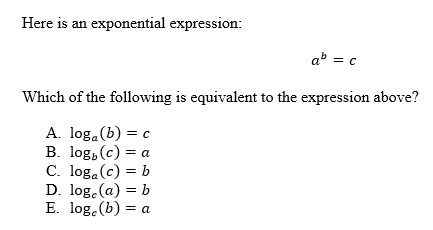#### Exponentials & Logarithms: College Algebra Lesson Plans Chapter Exam Instructions

Choose your answers to the questions and click 'Next' to see the next set of questions. You can skip questions if you would like and come back to them later with the yellow "Go To First Skipped Question" button. When you have completed the practice exam, a green submit button will appear. Click it to see your results. Good luck!

Support